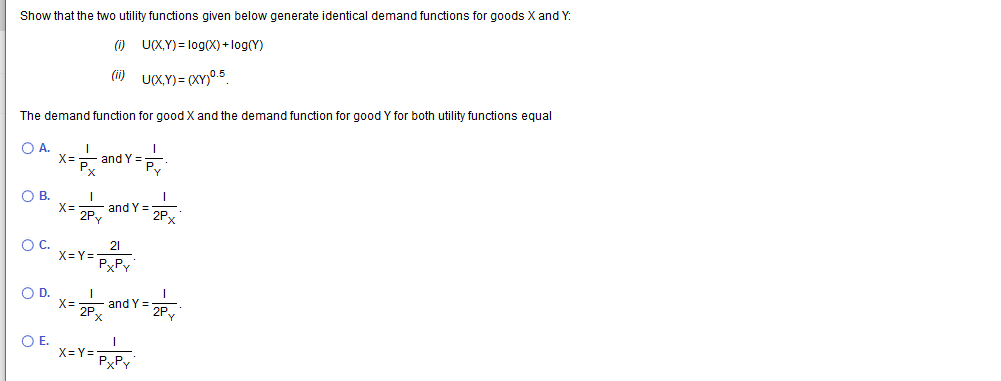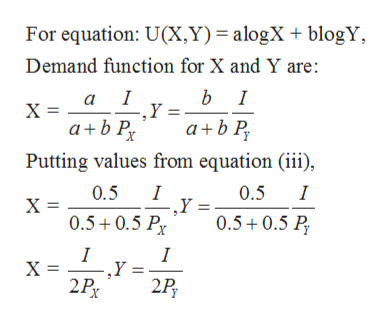# Show that the two utility functions given below generate identical demand functions for goods X and YUCXY) log(X)+log(YC) UCxY) (XY05The demand function for good X and the demand function for good Y for both utility functions equalO A.X=and YPvPxО В.Х-and Y2PvОс.X Y=21PXPYO D.Х-and Y2P.2PyОЕ.X= Y=РХPY

Question
109 viewshelp_outlineImage TranscriptioncloseShow that the two utility functions given below generate identical demand functions for goods X and Y UCXY) log(X)+log(Y C) UCxY) (XY05 The demand function for good X and the demand function for good Y for both utility functions equal O A. X= and Y Pv Px О В. Х- and Y 2Pv Ос. X Y= 21 PXPY O D. Х- and Y 2P. 2Py ОЕ. X= Y= РХPY fullscreen
check_circle

Step 1

Two utility functions are given which are:

Step 2

Instead of using equation (ii), monotonic transformation of equation (ii) can be done as follows:

Step 3

Now, equations (i) and (iii) are identical equations, so their demand functions will also be identi...help_outlineImage TranscriptioncloseFor equation: U(X,Y) = alogX + blogY Demand function for X and Y are: b I , Y b P a ab P а I Putting values from equation (ii), I ,Y 0.5+0.5 P 0.5 0.5 I Х- 0.5+0.5 P I ,Y 2Px I X 2P fullscreen

### Want to see the full answer?

See Solution

#### Want to see this answer and more?

Solutions are written by subject experts who are available 24/7. Questions are typically answered within 1 hour.*

See Solution
*Response times may vary by subject and question.
Tagged in